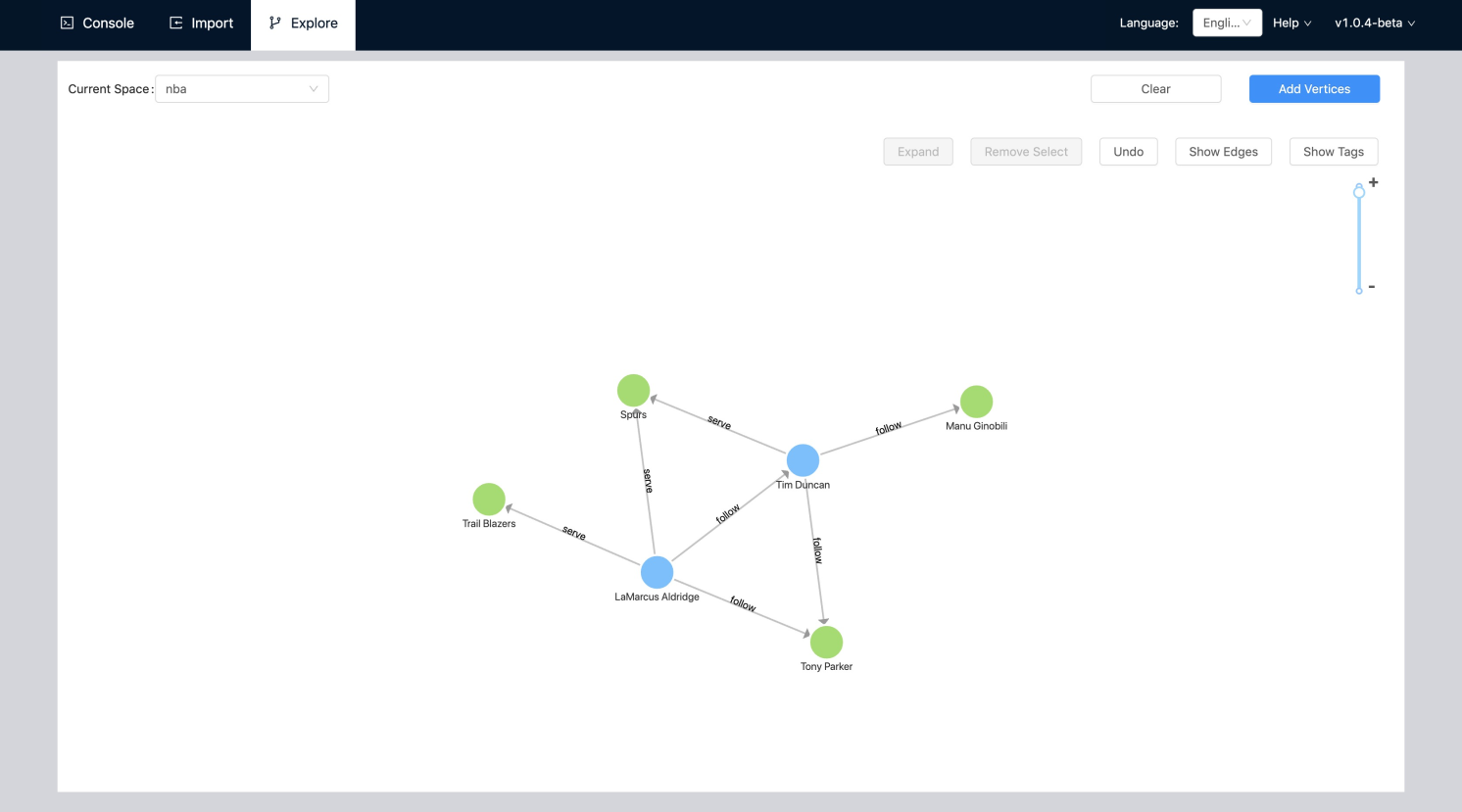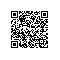# D3.js 力导向图的显示优化（二）- 自定义功能## 构建 D3.js 力导向图

this.force = d3
.forceSimulation()
// 为节点分配坐标
.nodes(data.vertexes)
// 连接线
// 整个实例中心
.force('center', d3.forceCenter(width / 2, height / 2))
// 引力
.force('charge', d3.forceManyBody().strength(-20))
// 碰撞力 防止节点重叠
.force('collide',d3.forceCollide().radius(60).iterations(2));## 支持删除任意选中功能

• 数据元素个数多于 DOM 元素个数
• 数据元素与 DOM 元素个数一样
• 数据元素个数少于 DOM 元素个数componentDidUpdate(prevProps) {
const { nodes } = this.props;
if (nodes.length < prevProps.nodes.length) {
const removeNodes = _.differenceBy(
prevProps.nodes,
nodes,
(v: any) => v.name,
);
removeNodes.forEach(removeNode => {
d3.select('#name_' + removeNode.name).remove();
});
} else {
this.labelRender(this.props.nodes);
}
}

## 支持按钮缩放功能

 <svg
width={width}
viewBox={0 0 ${width * (1 + scale)}${height * (1 + scale)}}
height={height}
>
{/*****/}
</svg>

 const { width, height } = this.props;
const scale = (100 - zoomSize) / 100;
const offsetX = width * (scale / 2);
const offsetY = height * (scale / 2);
// 操作节点边父元素 DOM <g/> 的偏移
d3.select(this.circleRef).attr(
'transform',
translate(${offsetX}${offsetY}),
);

## 结语使用钉钉扫一扫加入圈子
+ 订阅